## Friday, July 23, 2021

### DBDA2E R Scripts Updated for R 4.1

The R scripts that accompany DBDA2E have been updated so they work with R 4.1. Please go to the book's software page at
and scroll down to the bottom of that page to find the link to the zip file for the updated scripts.

I changed some scripts that use the R function read.csv() and relied on the old default of casting string vectors as factors. The default was changed in R 4.0, and the global option stringsAsFactors=TRUE no longer works for read.csv() in R 4.1.

## Friday, April 16, 2021

### Benchmark Bayes factors for uncertain prior model probability

I've posted a new manuscript titled "Uncertainty of prior and posterior model probability: Implications for interpreting Bayes factors." Here's a summary and examples to stimulate your interest.

Summary:

In most applications of Bayesian model comparison or Bayesian hypothesis testing, the results are reported in terms of the Bayes factor only, not in terms of the posterior probabilities of the models. Posterior model probabilities are not reported because researchers are reluctant to declare prior model probabilities, which in turn stems from uncertainty in the prior. Fortunately, Bayesian formalisms are designed to embrace prior uncertainty, not ignore it. This article provides

• novel formal derivations expressing the prior and posterior distribution of model probability
• a candidate decision rule that incorporates posterior uncertainty
• numerous illustrative examples
• benchmark BF’s using the uncertainty-based decision rule including benchmarks for a conventional uniform prior
• computational tools in R that are freely available at https://osf.io/36527/

I hope that this article provides both a conceptual framework and useful tools for better interpreting Bayes factors in all their many applications.

Examples:

Suppose you're doing a model comparison or hypothesis test, and you have a well-constructed Bayes factor of, say, BF=5. What do you conclude about the models or hypotheses? Your conclusion will depend on the posterior probabilities of the models, which in turn depend on the prior probabilities of the models. And what are the prior probabilities of the models? You're probably uncertain about the prior probabilities. Instead of pretending to have some specific point value for the prior model probabilities (as is usually done if it's done at all), we can represent the uncertainty as a distribution. The distribution of prior model probability becomes a distribution of posterior model probability, and we consider the entire distribution to decide about the models.

Notation: M1 is model 1, M2 is model 2. p(M1) is the prior probability of M1. BF is the Bayes factor for M1 relative to M2.

Figure 1, below, shows an example with a high-certainty (a.k.a., narrow, high-concentration) prior distribution at p(M1)=0.5. This prior distribution (see Panel A of Figure 1) represents a belief that the prior odds, p(M1)/p(M2), are almost certainly 50/50. When people assume any prior odds at all, this is the usual conventional for representing neutrality. Panel B shows the posterior distribution for BF=5 in favor of M1. Notice the probability of M1 has increased. Panel C shows the posterior distribution for BF=11.3, which is sufficient for the 95% HDI of the posterior distribution to exceed a decision criterion indicated by the vertical dashed line.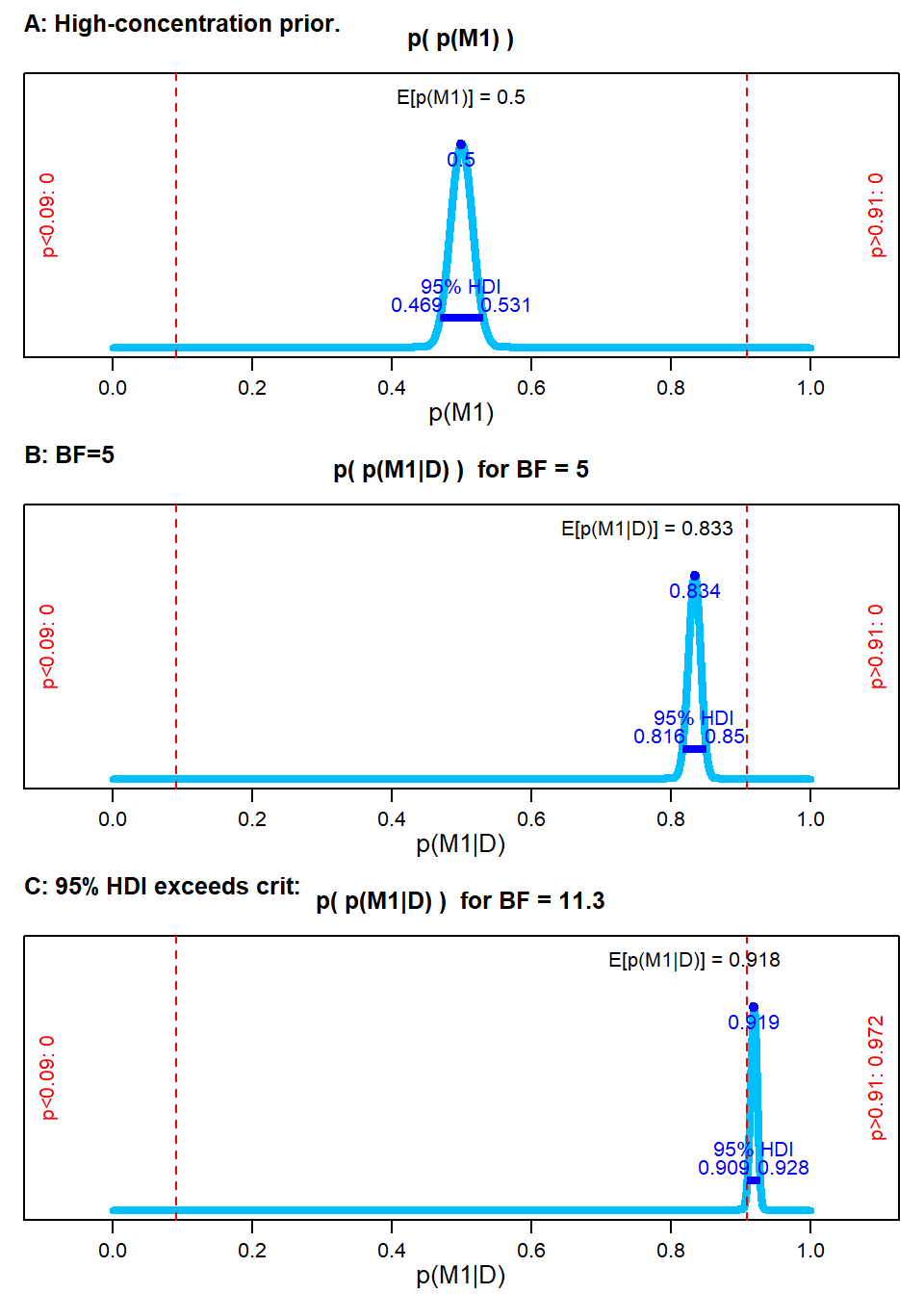Figure 1. A: High-certainty prior on p(M1). B: Posterior when BF=5. Posterior when BF=11.3.

Figure 2, by contrast, shows an example with a very uncertain (a.k.a., broad, low-concentration) prior distribution. This prior distribution (see Panel A of Figure 2) represents a much more typical state of prior knowledge, or at least is a much better representation of neutrality between models. Panel B shows the posterior distribution for BF=5. Notice it is very spread out. Panel C shows the posterior distribution for BF=38.9, which is sufficient for the 95% HDI of the posterior distribution to exceed a decision criterion (again indicated by the vertical dashed line). This BF might be treated as a benchmark when assuming a more realistic "neutral" prior for the model probabilities.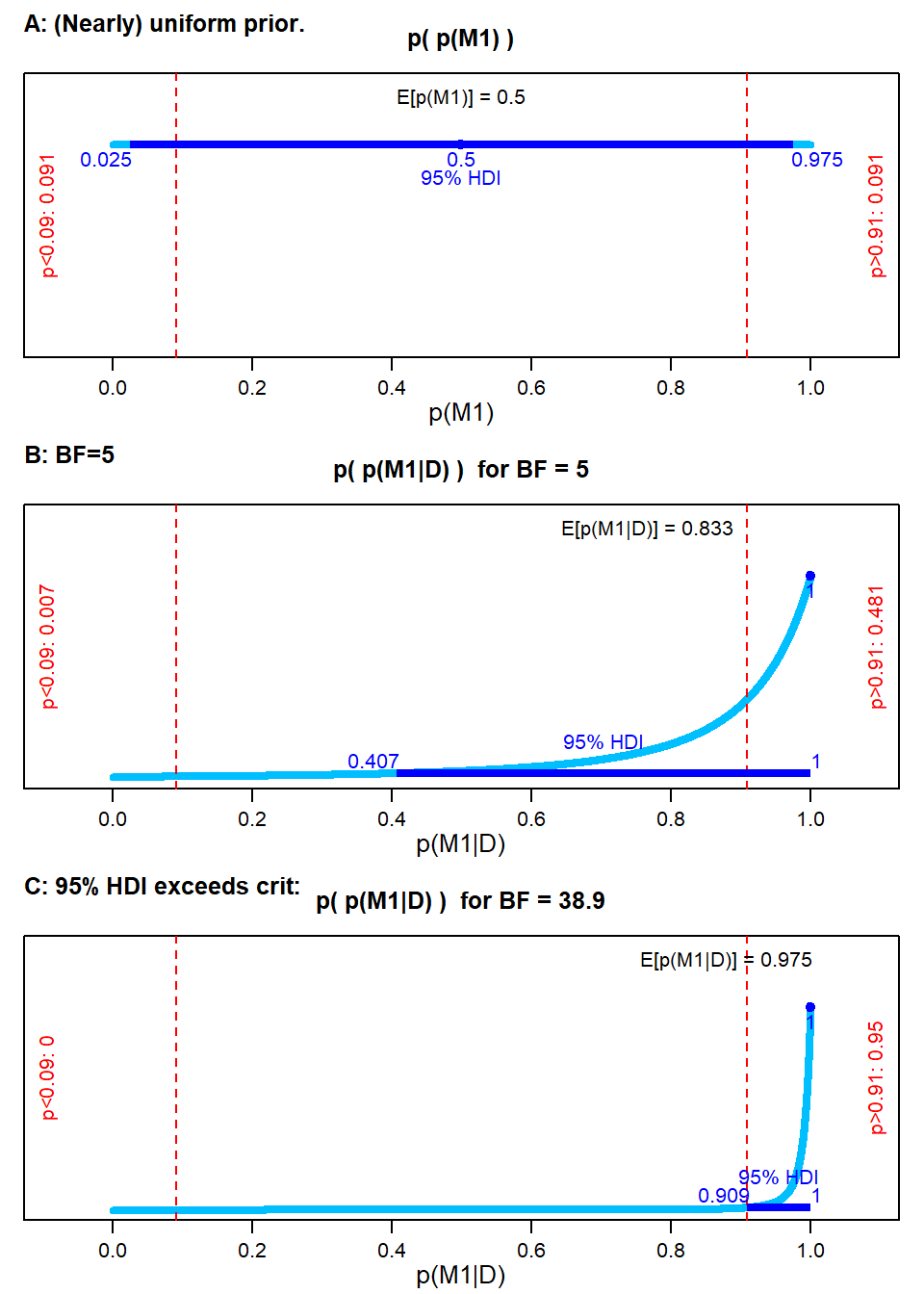Figure 2. A: Broad, uncertain prior on p(M1). B: Posterior when BF=5. C: Posterior when BF=38.9.

All the details are in the manuscript at https://osf.io/36527/. Please send me an email if you have comments!

## Sunday, October 25, 2020

### DBDA2E in brms and tidyverse

Solomon Kurz has been re-doing all the examples of DBDA2E with the brms package for ease of specifying models (in Stan) and with the tidyverse suite of packages for data manipulation and graphics. His extensive re-write of DBDA2E can be found here. It's definitely worth a look!

He has extensive re-writes of other books, too.

I've been meaning to make a post about this for ages, and have finally gotten around to it. Big thanks to Solomon Kurz!

## Monday, September 28, 2020

### Fixing a new problem in some DBDA2E scripts caused by a change in R 4.0.0

UPDATE: Scripts are now changed to work with R 4.1; see this blog post

The scripts that accompany DBDA2E have worked fine, "out of the box," for years. But recently some scripts have had problems. Why? R has changed. With R 4.0.0, various functions such as read.csv() no longer automatically convert strings to factors. Some DBDA2E scripts assumed the results of those functions contained factors, but if you're now using R 4.0.0 (or more recent) those scripts will balk.

So, what to do? Here's a temporary fix. When you open your R session, type in this global option:

options(stringsAsFactors = TRUE)

Unfortunately this option will eventually be deprecated. I'll have to modify every affected script and post updated versions. This will happen someday. I hope.

https://developer.r-project.org/Blog/public/2020/02/16/stringsasfactors/index.html

## Friday, August 14, 2020

### Need help finding corrigenda for DBDA2E

UPDATE: Now solved. Big thanks to Kent Johnson for re-constructing the table of corrigenda!

The host of the DBDA2E website (Google Sites), mandated a formatting change. Turns out the automatic reformatting mangled the table of Corrigenda. You can see it here:

I'd really like a properly formatted version!

Did you print or save or copy the previously formatted Corrigenda from the DBDA2E website sometime between October 2018 and August 2020? If so, please send it to me, and I'll attach it to the website.

(I got a version from the wayback machine at web.archive.org dated 2016, but there were subsequent modifications made until Sept 2018.)

Or, do you know of a way to re-format the mangled version so it appears properly?

Thanks!

## Thursday, May 28, 2020

### Teach (and learn) Bayesian and frequentist side by side

Teach (and learn) Bayesian and frequentist side by side: a talk and an app.

A talk explaining why that's a good idea:

(Talk delivered Saturday May 18, 2019.)

The interactive Shiny App with Bayesian and frequentist side by side: click HERE.
If you consider the app, especially for teaching, please let me know how it goes.

## Thursday, July 25, 2019

### Shrinkage in hierarchical models: random effects in lmer() with and without correlation

The goal of this post is to illustrate shrinkage of parameter estimates in hierarchical (aka multi-level) models, specifically when using lmer() with and without estimated correlation of parameters. The examples will show how estimates can differ when including correlation of parameters because of shrinkage toward the estimated correlation.

# Background

## Data structure for these examples

“It all begins with the data… ” I will create multiple panels of $\langle x , y \rangle$ data values, with $x$ and $y$ being continuous metric variables.
• For instance, each panel could be data from a student in a classroom, with each datum being performance on a standardized math exam, with $x$ being time and $y$ being performance. In this scenario, each student takes a novel variant of the test repeatedly across time. The times do not need to be the same for every student, and the number of tests do not need to be the same for every student. We are interested characterizing the performance trend of each panel (i.e., each student) and the overall trend across panels (i.e., for the class as a whole).
• As another example, each panel could be data from a distinct class within a school, with each datum being a particular student's exam performance (on the $y$ axis) and family income (on the $x$ axis). Again we are interested characterizing the trend of each panel (i.e., the relation of exam performance to family income within each classroom) and the overall trend across panels (i.e., the typical relationship of the variables across classrooms).
To illustrate robust shrinkage of panel estimates, each panel will have relatively few data points, and there will be relatively lots of panels. Graphs of the data will appear in analysis results, later.

Here (below) is the structure of the data. Notice there is an X variable, a Y variable, and a Panel variable. The panel variable is actually a nominal (categorical) value, even though it appears as a numerical index.
str( myData )

## 'data.frame':    208 obs. of  3 variables:
##  $X : num 0.4158 0.3795 0.0746 0.0588 0.4503 ... ##$ Y    : num  -0.864 -0.579 0.227 -1.604 -0.895 ...
##  \$ Panel: Factor w/ 35 levels "1","2","3","4",..: 1 1 1 1 1 1 2 2 2 3 ...


## Analysis models

For simplicity, each panel will be fit with a linear trend. The hierarchical (a.k.a. multi-level) models will also estimate the typical linear trend across panels.

Parameters for panels are subject to shrinkage in hierarchical models because the panel's linear trend is trying to conform simultaneously to (a) the data in its panel and (b) the typical trend across all panels. When there are lots of panels informing the typical trend, and only a small amount of data within a panel, then the panel estimates are strongly influenced by the typical trend across panels. This makes good sense: If you don't know much about a particular panel, your best estimate should take into account what's typical across many other similar panels.

For more background about shrinkage in hierarchical models, there are lots on online sources you can search, and you can see some of my previous writings on the topic:
I will first fit a line independently to each panel, without hierarchical structure. This analysis will show the estimated intercept and slope in each panel when there is no shrinkage.

I will then fit a hierarchical model that estimates a typical intercept and typical slope across panels, but does not estimate the correlation of the intercepts and slopes across panels. This model produces some shrinkage across panel estimates, but does not shrink the estimates toward a shared correlation across panels.

Finally, I will fit a hiearchical model that also estimates the correlation of intercepts and slopes across panels. This model shrinks the panel estimates so they also conform more strongly with the estimated correlation across panels.

For the non-hierarchical analysis, I will use lm() from the base stats package of R. For the hierarchical analyses, I will use lmer() from the lme4 package in R.

# Independent line for every panel

For this analysis, each individual panel is fit with its own line, separately from all other panels, using lm() on each panel. There is no hierarchical structure and no overall line estimated.

To make this analysis most analogous to subsequent analyses with lmer() the analyses should require all panels to have the same noise variance. But this is not done here, and actually the MLE coefficients are not affected in this case.

In principle, the analysis in this section would be like using lmer() with the formula y ~ 0 + (1+X||Panel), which specifies fitting lines within panels with no estimation of correlation across panels and no global parameters. But lmer() throws an error if that specification is attempted.
Here (below) are scatter plots of the data with the lm() fitted regression lines: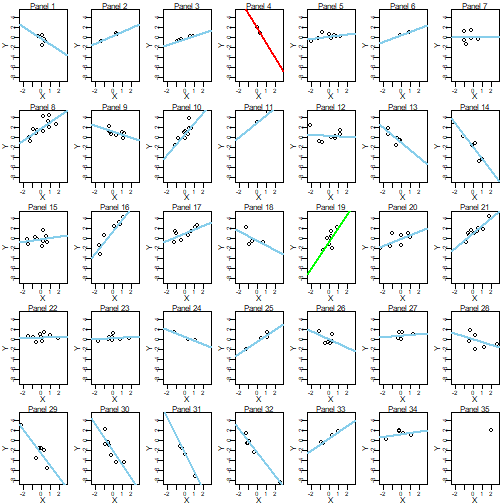Notice above:
• Two-point panels such as Panel 4 and Panel 11 have lines going exactly through the two points. This will not be the case in hierarchical models.
• The one-point Panel 35 has no regression line because it's undefined. This will not be the case in hierarchical models.
• Panels 4 and 19 are color-highlighted for easy comparison with subsequent analyses.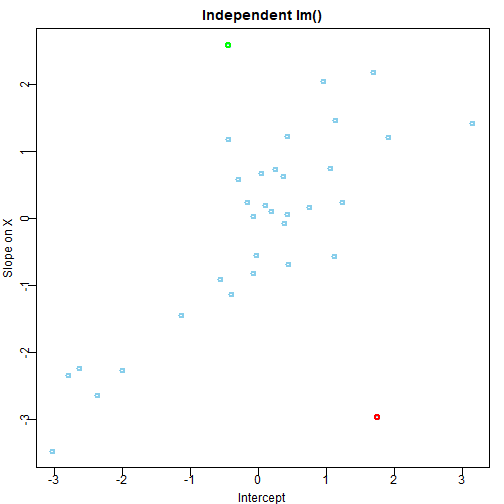Notice above:
• There is correlation of intercepts and slopes across panels (r=0.65), reflecting only how the data were generated, not any estimation of correlation in the model.
• There is a lot of variation in intercepts and slopes across panels relative to hierarchical (multi-level) models below. There will be less variation in hierarchical models, hence the term shrinkage.
• Panels 4 and 19 are color-highlighted for easy comparison across analyses.

# Random intercepts and slopes, but no estimated correlation

I'll use lmer() with the formula, Y ~ 1 + X + ( 1 + X || Panel ), which is equivalent to Y ~ 1 + X + ( (1|Panel) + (0+X|Panel) ). lmer() assumes we want to estimate correlations of parameters across panels unless we tell it not to by using a double vertical bar or by explicitly coding the separate effects.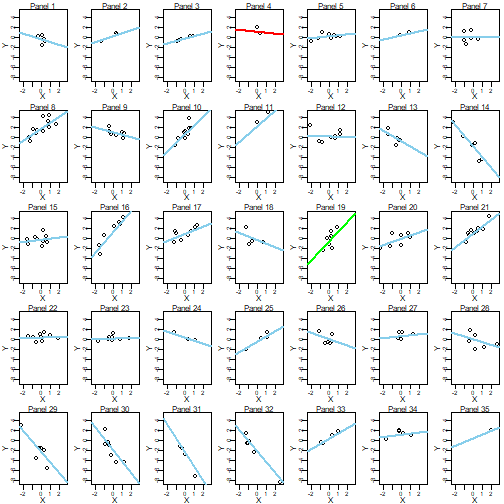Notice above:
• Two-point panels such as Panel 4 and Panel 11 have lines not going exactly through the two points. This is because the line is trying to conform simultaneously to the data in the panel and what's typical across panels, as estimated by this particular hierarchical model.
• The one-point Panel 35 has a regression line despite having only a single point. This is because the line is generated by what's typical across panels, influenced a bit by the single data point in the panel.
• Panels 4 and 19 are color-highlighted for easy comparison with across analyses.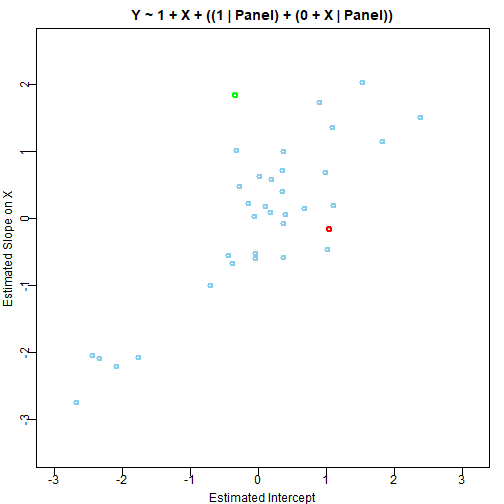Notice above:
• There is correlation of intercepts and slopes across panels (r=0.814), but this reflects only how the data were generated and the separate shrinkage of intercepts and slopes, without any shrinkage from estimation of correlation.
• There is less variation in intercepts and slopes across panels relative to the previous, non-hierarchical analysis, hence the term shrinkage. Specifically, the range of slopes across panels in the non-hierarchical model was -3.48, 2.59 but the range of slopes in this hierarchical model is -2.74, 2.03.
• Panels 4 and 19 are color-highlighted for easy comparison across analyses.

# Random intercepts and slopes, with estimated correlation

Here I use lmer() with forumla Y ~ 1 + X + ( 1 + X | Panel ). Notice the single vertical bar before Panel, so lmer() estimates the correlation of parameters across panels by default.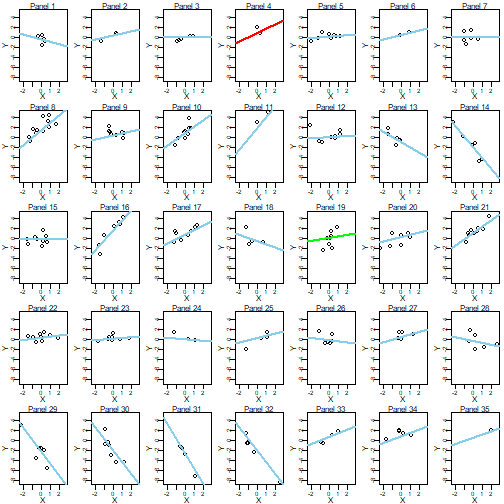Notice above:
There is even more shrinkage than in the previous model, because now the lines in each panel are also “trying” to conform to the typical correlation of intercept and slope across panels. Notice in particular the color-coded lines in panels 4 and 19.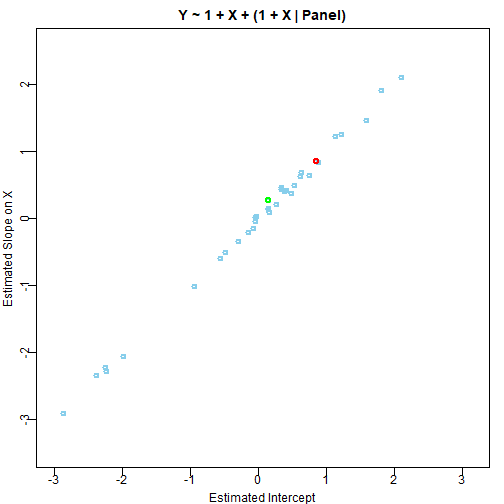Notice above:
There is a strong correlation between the estimated slopes and intercepts (r=0.998). Here the correlation is estimated and there is shrinkage of estimates toward that correlation, and the correlation is stronger than the previous model because the estimates are shrunken toward that correlation.

# What about the higher-level, "fixed" effects?

The higher-level intercept and slope are at the means of the panel intercepts and panel slopes, and those overall means are essentially the same across these analyses.

# Conclusion

Hopefully these examples have helped to illustrate shrinkage in hierarchical models, and specifically the extra shrinkage introduced by estimating correlation across parameters.